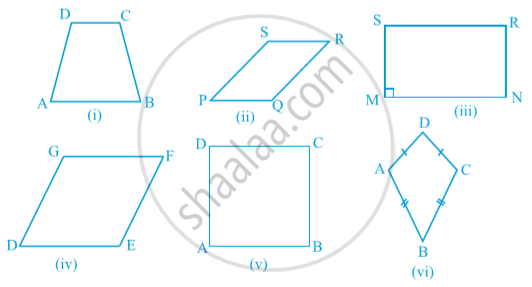Share

Course

#### textObserve that :
⦁ One pair of opposite sides of quadrilateral ABCD in Fig. (i) namely, AB and CD are parallel. You know that it is called a trapezium.
⦁  Both pairs of opposite sides of quadrilaterals given in Fig. (ii), (iii) , (iv) and (v) are parallel. Recall that such quadrilaterals are called parallelograms.
⦁  In parallelogram MNRS of Fig. (iii), note that one of its angles namely ∠ M is a right angle.  It is called a rectangle.
⦁  The parallelogram DEFG of Fig. (iv) has all sides equal and we know that it is called a rhombus.
⦁ The parallelogram ABCD of Fig. (v) has ∠ A = 90° and all sides equal; it is called a square.
⦁  In quadrilateral ABCD of Fig. (vi), AD = CD and AB = CB i.e., two pairs of adjacent sides are equal. It is not a parallelogram. It is called a kite.

Note that a square, rectangle and rhombus are all parallelograms.
⦁ A square is a rectangle and also a rhombus.
⦁ A parallelogram is a trapezium.
⦁  A kite is not a parallelogram.
⦁  A trapezium is not a parallelogram (as only one pair of opposite sides is parallel in a trapezium and we require both pairs to be parallel in a parallelogram).
⦁ A rectangle or a rhombus is not a square.

If you would like to contribute notes or other learning material, please submit them using the button below.